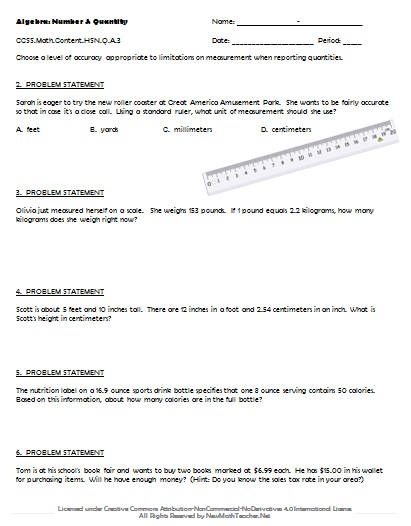# Engaging Math Lessons.

Please browse our selection of mathematics lessons sorted by subject and current common core standards for improving math achievement for every student.

## Traditional Pathway: Algebra 1

Below are a comprehensive set of lesson plans and worksheets for Algebra 1 that correspond to the given Common Core concept and help students solve real-world problems. Lesson previews provide a quick glimpse at the provided materials.

• ### Concept:

Number & Quantity: The Real Number System
• Common Core Standard:CCSS.Math.Content.HSN.RN.A.1
• Description: Explain how the definition of the meaning of rational exponents follows from extending the properties of integer exponents to those values, allowing for a notation for radicals in terms of rational exponents.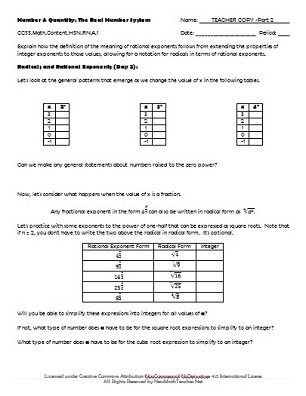• ### Concept:

Number & Quantity: The Real Number System
• Common Core Standard:CCSS.Math.Content.HSN.RN.A.2
• Description: Rewrite expressions involving radicals and rational exponents using the properties of exponents.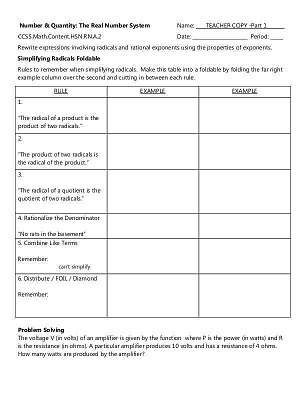• ### Concept:

Number & Quantity: The Real Number System
• Common Core Standard:CCSS.Math.Content.HSN.RN.B.3
• Description: Explain why the sum or product of two rational numbers is rational; that the sum of a rational number and an irrational number is irrational; and that the product of a nonzero rational number and an irrational number is irrational.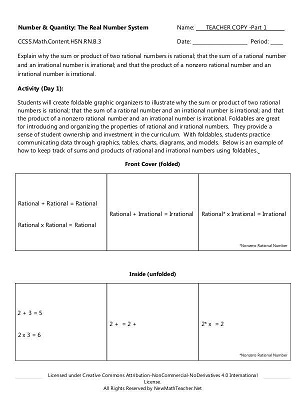• ### Concept:

Number & Quantity: Quantities
• Common Core Standard:CCSS.Math.Content.HSN.Q.A.1
• Description: Use units as a way to understand problems and to guide the solution of multi-step problems; choose and interpret units consistently in formulas; choose and interpret the scale and the origin in graphs and data displays.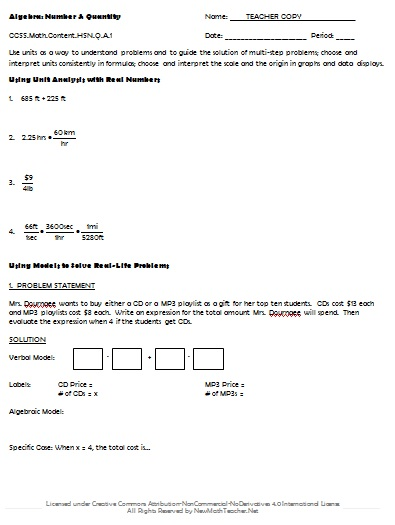• ### Concept:

Number & Quantity: Quantities
• Common Core Standard:CCSS.Math.Content.HSN.Q.A.2
• Description: Define appropriate quantities for the purpose of descriptive modeling.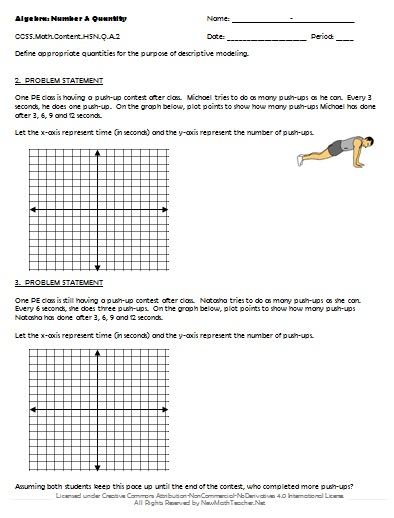• ### Concept:

Number & Quantity: Quantities
• Common Core Standard:CCSS.Math.Content.HSN.Q.A.3
• Description: Choose a level of accuracy appropriate to limitations on measurement when reporting quantities.